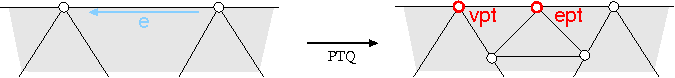### Definition

A stencil determines a source neighborhood whose points contribute to the position of a refined point. The geometry mask of a stencil specifies the computation on the nodes of the stencil. Loop_mask_3<Polyhedron_3> implements the geometry masks of Loop subdivision on a triangulated Polyhedron_3<Cartesian>.

### Parameters

The full template declaration of Loop_mask_3<Polyhedron_3> states one template parameter:

 template < class Polyhedron_3> class Loop_mask_3;

The only parameter requires a Polyhedron_3 as the argument. The Polyhedron_3 should be specialized with the Cartesian kernel, which defines the Point_3 for the vertices.

### Creation